# Surface Area Of Cones Worksheet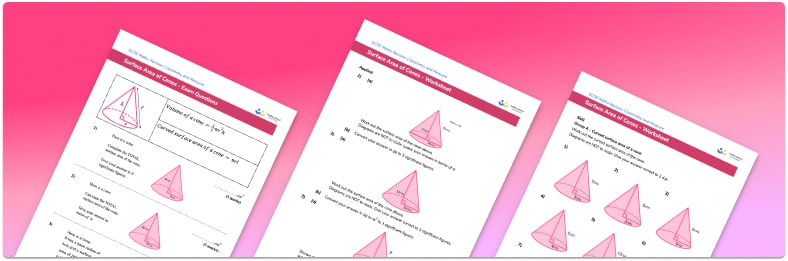• Section 1 of the surface area of a cone worksheet contains 27 skills-based surface area of cones questions, in 3 groups to support differentiation
• Section 2 contains 4 applied surface area of cones questions with a mix of word problems and deeper problem solving questions
• Section 3 contains 3 foundation and higher level GCSE exam style surface area of cones questions
• Answers and a mark scheme for all surface area of cones questions are provided
• Questions follow variation theory with plenty of opportunities for learners to work independently at their own level
• All questions created by fully qualified expert secondary maths teachers
• Suitable for GCSE maths revision for AQA, OCR and Edexcel exam boards

• This field is for validation purposes and should be left unchanged.

You can unsubscribe at any time (each email we send will contain an easy way to unsubscribe). To find out more about how we use your data, see our privacy policy.

### Surface area of cones at a glance

Surface area is a measure of the total area of all of the faces of a 3D shape. A cone has two faces, a circular base and a curved face. We know that the area of a circle is given by πr^2  where r is the radius of the circle. To find the curved surface area of a cone, also called the lateral surface area, we use the formula \text{curved surface area} = πrl where r is the radius of the base and l is the slant height of the cone. Note that the slant height is different to the perpendicular height of the cone.

To find the total surface area of the cone, we add together the area of the two faces to get \text{Surface Area} = πrl \times π(r^2) .

Surface area is measured in square units, such as cm^2  or mm^2 . We may be asked to give an answer in terms of pi ( π ) or to round to a given number of significant figures or decimal places.

Looking forward, students can then progress to additional surface area worksheets and other geometry worksheets, for example a simplifying expressions worksheet or simultaneous equations worksheet.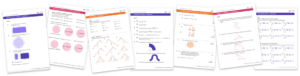For more teaching and learning support on Geometry our GCSE maths lessons provide step by step support for all GCSE maths concepts.

## Related worksheets

Surface Area Of A Triangular Prism Worksheet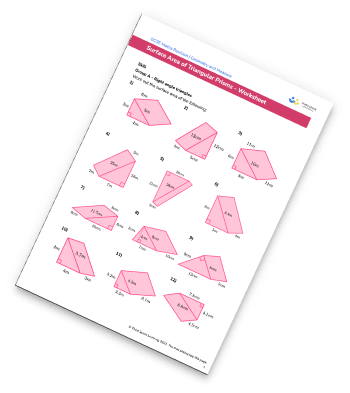Surface Area Of Cylinders Worksheet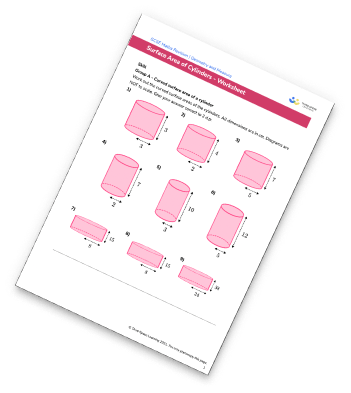Surface Area Of A Cuboid Worksheet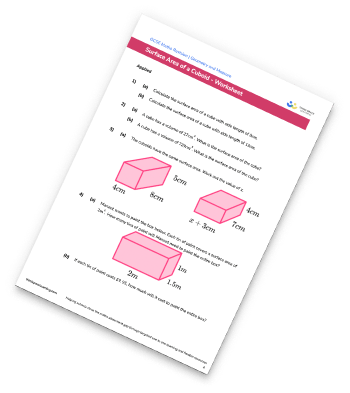Surface Area Of Prisms Worksheet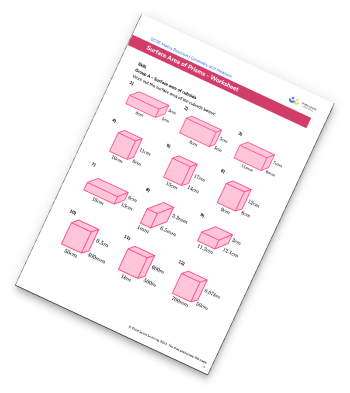## Do you have KS4 students who need more focused attention to succeed at GCSE?There will be students in your class who require individual attention to help them succeed in their maths GCSEs. In a class of 30, it’s not always easy to provide.

Help your students feel confident with exam-style questions and the strategies they’ll need to answer them correctly with our dedicated GCSE maths revision programme.

Lessons are selected to provide support where each student needs it most, and specially-trained GCSE maths tutors adapt the pitch and pace of each lesson. This ensures a personalised revision programme that raises grades and boosts confidence.

Find out more